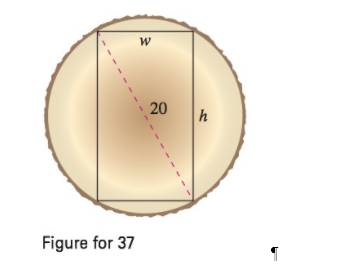Chapter 4.7, Problem 37ECalculus: Early Transcendental Fun...

7th Edition
Ron Larson + 1 other
ISBN: 9781337552516

Solutions

Chapter
SectionCalculus: Early Transcendental Fun...

7th Edition
Ron Larson + 1 other
ISBN: 9781337552516
Textbook Problem

Beam Strength A wooden beam has a rectangular cross section of height h and width w (see figure). The strength. S' of the beam is directly proportional to the width and the square of the height. What are the dimensions of the strongest beam that can be cut from a round log of diameter 20 inches? (Hint: S = k h 2 w , where k is the proportionality constant.)To determine

To calculate: The dimensions of the strongest beam cut from a log that has a diameter of 20 inches.

Explanation

Given:

The log has diameter 20 inches and the strength S of the beam is given by the formula:

S=kh2w

Here, w is the width and h the height.

Formula used:

For a function f that is twice differentiable on an open interval I, if f'(c)=0 for some c, then,

If f''(c)>0 the function f has relative minima at c if f''(c)<0 the function f has relative maxima at c.

Calculation:

The diameter would be the hypotenuse of the rectangle with its two other sides as the width and the height.

By Pythagoras Theorem,

w2+h2=202h2=400w2h=400w2

Substitute this in the formula for strength to obtain:

S(w)=k(400w2)2w=k(400w2)w=k(400ww3)

Differentiate this with respect to w and equate it to 0

Still sussing out bartleby?

Check out a sample textbook solution.

See a sample solution

The Solution to Your Study Problems

Bartleby provides explanations to thousands of textbook problems written by our experts, many with advanced degrees!

Get Started

Find more solutions based on key concepts